# A spring with a force constant of 20 newtons per meter is known to obey Hooke's Law. The spring is attached to a mass of 5 kilograms and placed on a horizontal, frictionless surface. The mass is displaced 3 meters from its equilibrium position and released. What is the kinetic energy of the mass as it passes through its equilibrium position?

Question
1 views

A spring with a force constant of 20 newtons per meter is known to obey Hooke's Law. The spring is attached to a mass of 5 kilograms and placed on a horizontal, frictionless surface. The mass is displaced 3 meters from its equilibrium position and released. What is the kinetic energy of the mass as it passes through its equilibrium position?

check_circle

Step 1

Introduction- The principle of the conservation of mechanical energy states that the total mechanical energy in a system (i.e., the sum of the potential plus kinetic energies) remains constant as long as the only forces acting are conservative forces (i.e., spring force, gravitational force).

given- Force constant of a spring,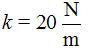Mass,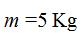Displacement,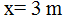Determine the kinetic energy of the mass at the equilibrium position of a spring

Step 2

From the conservation of mechanical energy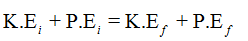When the mass displaced 3 meters from its equilibrium position initial kinetic energy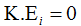And initial potential energy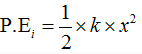When the mass passes through the equilibrium position of spring final kinetic energy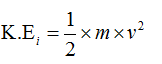And final potential energy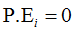Step 3

From the conservation of mechanical energy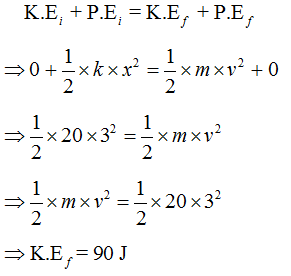...

### Want to see the full answer?

See Solution

#### Want to see this answer and more?

Solutions are written by subject experts who are available 24/7. Questions are typically answered within 1 hour.*

See Solution
*Response times may vary by subject and question.
Tagged in